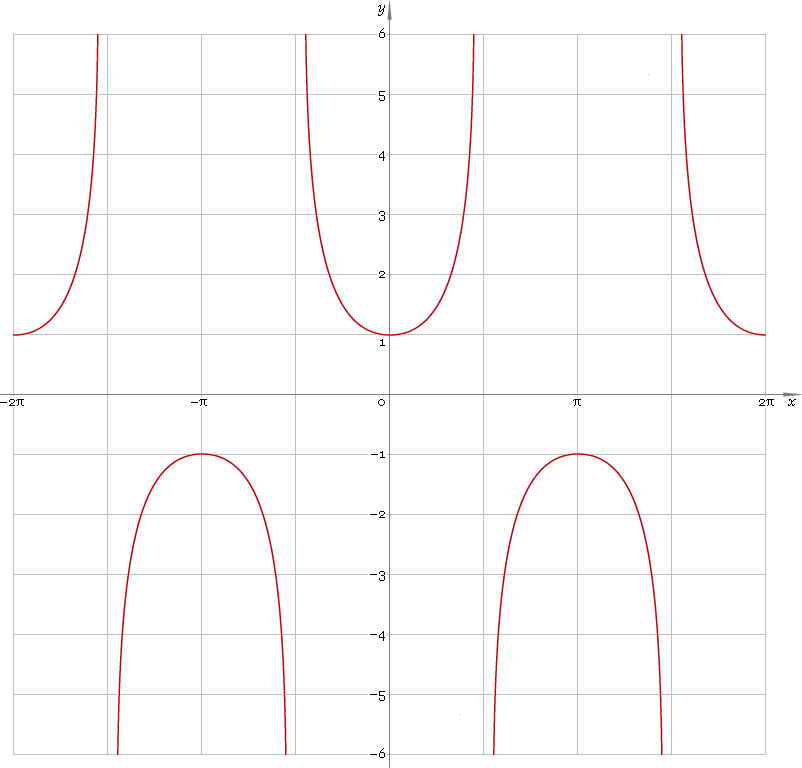The Art of Mathematics

# sec — trigonometric secant function

## 1. Definition

Secant of the angle is ratio of the hypotenuse to adjacent leg.

## 2. Graph

Secant is 2π periodic function defined everywhere on real axis, except its singular points π/2 + πn, n = 0, ±1, ±2, ... — so function domain is (−π/2 + πn, π/2 + πn), n∈N. Its stalactite-stalagmite graph is depicted below — fig. 1.Fig. 1. Graph of the secant function y = secx.

Function codomain is all real axis with gap in the middle: (−∞, −1]∪[1, +∞).

## 3. Identities

Base:

csc−2φ + sec−2φ = 1

and its consequences:

secφ = ±1 /√(1 − sin2φ)
secφ = ±√(1 + tan2φ)
secφ = ±√(1 + cot2φ) / cotφ
secφ = ±cscφ /√(csc2φ − 1)

By definition:

secφ ≡ 1 /cosφ

Properties — symmetry, periodicity, etc.:

sec−φ = cscφ
secφ = sec(φ + 2πn), where n = 0, ±1, ±2, ...
secφ = −sec(π − φ)
secφ = −sec(π + φ)
secφ = csc(π/2 + φ)

Sum of angles:

sec(φ + ψ + χ) = secφ secψ secχ / (1 − tanφ tanψ − tanφ tanχ − tanψ tanχ)

Some angles:

Angle φValue secφ
01
π/12√6 − √2
π/10√(50 − 10√5) /5
π/8√(2 − √2)
π/62√3 /3
π/5√5 − 1
π/4√2
3π/10√(50 + 10√5) /5
π/32
3π/8√(4 + 2√2)
2π/5√5 + 1
5π/12√6 + √2
Table 1. Secant for some angles.

## 4. Derivative and indefinite integral

Secant derivative:

sec′x = secx tanx ≡ sinx /cos2x

Indefinite integral of the secant:

∫ secx dx = ln|secx + tanx| + C

where C is an arbitrary constant.

## 5. How to use

To calculate secant of the number:

``sec(−1);``

To get secant of the complex number:

``sec(−1+i);``

To get secant of the current result:

``sec(rslt);``

To get secant of the angle φ in calculator memory:

``sec(mem[φ]);``

## 6. Support

Trigonometric secant of the real argument is supported in free version of the Librow calculator.

Trigonometric secant of the complex argument is supported in professional version of the Librow calculator.